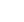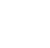# Faculty of Mechanics and Mathematics

Price: 9669 EUR
Contact GSP-1, Leninskie Gory, Moscow, 119991, Russian Federation

## Description

Mathematics has always been the basis of natural science and, together with mechanics, it is the basis of all engineering sciences and the main means to investigate laws of the Universe.

Lectures on Mathematics have been given at Moscow University since its opening in 1755. The Department of Physics and Mathematics was first mentioned in the 1804 Charter.

By the beginning of the 20th century the Moscow University school of mathematics became world-known. Its success was due to the work of its founder D. F. Yegorov and his pupils and followers N. N. Luzin, I. I. Privalov, and V. V. Stepanov. The Moscow University school of mechanics owed its fame to the works of N. E. Zhukovsky and S. A. Chaplygin.

In 1933 Moscow University was reorganized, several new faculties were established, among them the Faculty of Mechanics and Mathematics. A great role in its establishment and further development belonged to Corresponding member of the Academy of Sciences of the USSR V. V. Golubev, academicians I. G. Petrovsky, A. N. Kholmogorov, P. S. Aleksadrov.

Our graduates have contributed to the development of academic science in Russia, at the same time many members of the Academy of Sciences have taught at the faculty. At present there are 7 academicians and 6 corresponding members of the Russian Academy of Sciences heading our departments and laboratories.

Traditionally, our students are taught not the methods to solve a particular problem but the ability to think and make decisions independently and to extract and analyze information from various sources. That is why our graduates are able to go into any problem in almost every professional sphere: from IT to finance, from business management to politics.

Nowadays the role of mathematics in the system of human knowledge has grown considerably. Mathematical methods are applied to design nuclear reactors, to study the structure of crystals and molecules of chemical substances, to predict the location of mineral deposits, to forecast the weather. Mathematical methods can help to analyze economic changes and optimize the management of economy, to diagnose illnesses, to decipher ancient manuscripts, to substantiate sociological survey results. Mathematical methods are seen as the basis for IT, which is said to have changed the modern world. The most abstract branches of mathematics, such as mathematical logic, functional analysis, topology, modern algebraic theories, have recently found a number of practical applications too.

The system of mathematical sciences itself has undergone dramatic changes. New mathematical disciplines have appeared, for example, homological algebra, differential geometry, computational geometry. Such fields of mathematics as mathematical logic, probability theory, optimal control theory have been seriously changed.

MSU scientists have greatly contributed to the most important areas of mathematics. MSU school of mathematics is a recognized authority in the world and a leading centre of mathematical sciences in Russia.

Mechanics is a cornerstone of engineering. Scientists investigate and develop mathematical models of motion and interaction of bodies and particles as well as mass interaction, they study mechanical characteristics of various media. Without dealing with a particular design, specialists in mechanics create a theoretical basis to apply in other sciences, in engineering and in industry. The achievements of MSU school of mechanics are world-recognized, they have influenced the development of modern mechanical engineering.

A variety of applications for mathematical knowledge, including the most recently developed branches of theoretical and calculus mathematics, is typical of modern mechanics. The numerical experiment became one of the most efficient methods for mechanical engineers to use. Nevertheless, the relationship between mechanics and mathematics is not one-sided. Mechanics stimulates the development of numerous branches of mathematics, formulating problems that mathematics is to find solutions to. This fruitful interaction accounts for these sciences being taught at the same faculty at MSU.

The Faculty of Mechanics and Mathematics has two divisions: the Division of Mechanics the Division of Mathematics. We offer 6-year Specialist’s Degree programs in Mechanics and Mathematics, the latter division offering a major program in Mathematical Methods in Economics as well.

The Division of Mathematics includes Department of Mathematical Analysis, Department of Higher Algebra, Department of Higher Geometry and Topology, Department of Probability Theory, Department of General Control Problems, Department of Number Theory, Department of Differential Equations, Department of Theory of Functions and Functional Analysis, Department of Mathematical Logic and Theory of Algorithms, Department of Mathematical Statistics and Random Processes, Department of Discrete Mathematics, Department of Calculus Mathematics, Department of General Topology and Geometry, Department of Mathematical Theory of Intelligent Systems, Department of Differential Geometry and Its Applications, Department of the Theory of Dynamical Systems, Department of Mathematical and Numerical Methods of Analysis.

The Division of Mechanics includes: Department of Theoretical Mechanics and Mechatronics, Department of Aeromechanics and Gas Dynamics, Department of Theory of Elasticity, Department of Hydromechanics, Department of Applied Mechanics and Control, Department of Gas and Wave Dynamics, Department of Theory of Plasticity, Department of Mechanics of Composite Materials, Department of Calculus Mechanics.

Our curriculum covers all modern areas of Mathematics and Mechanics. Although the scope differs, a number of disciplines are included in the curricula of both our divisions: Mathematical Analysis, Algebra, Analytic Geometry, Differential Equations, Mathematical Physics Equations, Function Theory and Functional Analysis, Probability Theory, Mathematical Statistics, Calculus Methods, Programming, Complex Analysis, Differential Geometry and Topology, Theoretical Mechanics, Mechanics of Continua, Physics, Mathematical Methods in Economics, Operations Research. All our students also study History, Methodology of Mathematics and Mechanics, they have core courses of the Humanities and the English language.

The curriculum of the Mathematics Division includes courses of natural sciences, applied science and economics. Students of the Mechanics Division acquire their practical experience in the laboratories of the MSU Institute of Mechanics.

In their 1st and 2nd year our students study the core subjects of the curriculum. In their 3rd year they choose their major areas and a department where an adviser directs their research work. Students and postgraduate students publish the results of their research in leading journals, they participate in various competitions and contests in Mathematics, Computer Science, Robot Technology, etc, both in Russia and abroad. For over 10 years our teams have taken part in the International Collegiate Programming Contest, having been among the finalist many times and won golden medals twice (2003 and 2005).

The Faculty of Mechanics and Mathematics together with the MSU Institute of Mechanics have organized the Students’ Contests in Robot Technology, making it a tradition. The participants of the contests can implement their mathematical models in practice, going all the way from formulating a theoretical problem to constructing the robot.

The Faculty of Mechanics and Mathematics also comprises the Department of Applied Research, including the Educational Scientific Centre and the Laboratory of Artificial Intelligence, the Laboratory of Dynamics of Deformable Media, the Laboratory of Space Navigation and Systems, the Laboratory of Mathematical Modeling for Dynamic Systems Simulation, the Laboratory of Applied Mathematical Analysis.

There are scientific laboratories at the departments: the Laboratory of Calculus Methods, the Laboratory of Mathematical Statistics, the Laboratory of Probability Theory, the Laboratory of Calculus Methods for Probability and Statistics, the Laboratory of Computer Modeling, the Laboratory of Large Random Systems, the Laboratory of Computer Methods in Natural Sciences and Humanities, the Laboratory of Operator Models and Spectral Theory, the Department of History and Methodology of Mechanics and Mathematics and the Department of Methods of Teaching Elementary Mathematics. Undergraduates as well as post-graduate students are involved in research work carried our by the laboratories.

The Faculty of Mechanics and Mathematics is affiliated with the MSU Institute of Mechanics whose research work embraces all the main areas of Mechanics. The Institute’s experimental facilities are used by Mechanics students both for classes and research.

Our graduates work in research institutes, in various companies and in banks, in laboratories and computation centers, in institutions of higher education and schools, successfully solving both theoretical and applied problems. Around a third of our graduates go on to our graduate school, doing research for their Candidate’s or Doctor’s degrees in Physics and Mathematics. Degrees awarded by the MSU Faculty of Mechanics and Mathematics are recognized all over the world.

## Specific details

Category of Education Technology and Engineering, Education

## University

Lomonosov Moscow State University

## Location

Russian Federation
GSP-1, Leninskie Gory, Moscow, 119991, Russian Federation

There are no comments posted here yetinfo@educom.net
 About Terms and ConditionsPrivacy PolicyRankingsAccreditations